# Cylindrical tank 2

If a cylindrical tank with volume is used 12320cm raised to the power of 3 and base 28cm is used to store water. How many liters of water can it hold?

V =  12.32 l

### Step-by-step explanation: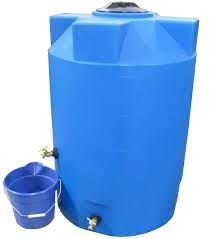Did you find an error or inaccuracy? Feel free to write us. Thank you!

Showing 1 comment:Math student
how to mesure h an lTips to related online calculators
Do you know the volume and unit volume, and want to convert volume units?

## Related math problems and questions:

• Water tankThe water tank has a cylindrical shape with a base diameter of 4.2 m and is 80 cm deep. How many minutes will take fill it 10 cm below the edge of the tank if water flowing 2 liters per second?
• Liters in cylinderDetermine the height at which level 24 liters of water in a cylindrical container having a bottom diameter 36 cm.
• Water tankWhat is the height of the cuboid-shaped tank with the bottom dimensions of 80 cm and 50 cm if the 480 liters of water reaches 10 cm below the top?
• Tank and waterWe poured a cylindrical tank with 3.5 liters of water. If the tank base diameter is 3 dm, how height is the water level in?
• A cylindrical tankA cylindrical tank can hold 44 cubic meters of water. If the radius of the tank is 3.5 meters, how high is the tank?
• Half-filled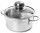A cylindrical pot with a diameter of 24 cm is half-filled with water. How many centimeters will the level rise if we add a liter of water to it?
• Collect rain waterThe garden water tank has a cylindrical shape with a diameter of 80 cm and a height of 12 dm. How many liters of water will fit into the tank?
• Mike built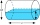Mike built a cylindrical water tank with a radius of about 1.8 m and height of 2 meters. How many liters of water will it hold?
• Cylindrical tank9.6 hl of water is poured into a cylindrical tank with a bottom diameter of 1.2 m. What height in centimeters does the water reach?
• Water tankWater tank shape of cuboid has dimensions of the bottom 7.5 meters and 3 meters. How high will reach the water in the tank will flow 10 liters of water per second and the inflow will be open 5/6 hour? (Calculate to one decimal place and the results give i
• The tankThe tank has 1320 liters of water. The tank has the shape of a prism, its base is an rectangle with sides a = 0,6 m and b = 1,5 m. How high does the water level reach in the tank?
• WaterInto a full cylindrical tank high 3 m with a base radius of 2.5 m, we insert cuboid with dimensions 1.7 m, 1.3 m, 1.9 m. How many liters of water will overflow out?
• AquariumAquarium is rectangular box with square base containing 76 liters of water. Length of base edge is 42 cm. To what height the water level goes?
• Water overflow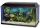A rectangular container that has a length of 30 cm, a width of 20 cm, and a height of 24 cm is filled with water to a depth of 15 cm. When an additional 6.5 liters of water are poured into the container, some water overflows. How many liters of water over
• Water tank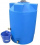The block-shaped tank is 2.5 m long, 100 cm wide and 12 dm high. In how many minutes will it be filled with water to two thirds if 40 liters of water flow into the tank per minute?
• AquariumHow many liters of water can fit in an aquarium measuring 30, 15 and 20 cm?
• Two vases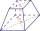Michaela has two vases in her collection. The first vase has the shape of a cone with a base diameter d = 20 cm; the second vase has the shape of a truncated cone with the lower base d1 = 25 cm and with the diameter of the upper base d2 = 15 cm. Which vas